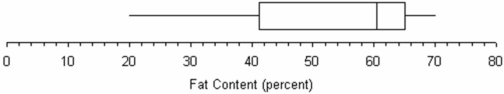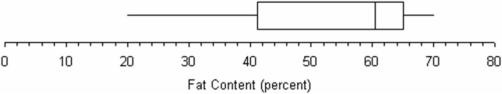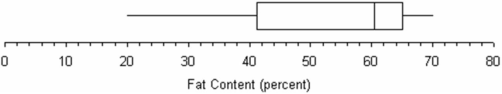/
/
/
21.Referring to this box plot of ice cream fat content,
Not my Question
Flag Content

# Question : 21.Referring to this box plot of ice cream fat content, : 1788690

21.Referring to this box plot of ice cream fat content, the mean would exceed the median.22.Referring to this box plot of ice cream fat content, the skewness would be negative.23.Referring to this graph of ice cream fat content, the second quartile is about 61.24.The range as a measure of variability is very sensitive to extreme data values.

25.In calculating the sample variance, the sum of the squared deviations around the mean is divided by n - 1 to avoid underestimating the unknown population variance.

26.Outliers are data values that fall beyond ±2 standard deviations from the mean.

27.The Empirical Rule assumes that the distribution of data follows a normal curve.

28.The Empirical Rule can be applied to any distribution, unlike Chebyshev'stheorem.

29.When applying the Empirical Rule to a distribution of grades, if a student scored one standard deviation below the mean, then she would be at the 25th percentile of the distribution.

30.Kurtosis cannot be judged accurately by looking at a histogram.

31.A platykurtic distribution is more sharply peaked (i.e., thinner tails) than a normal distribution.

32.A leptokurtic distribution is more sharply peaked (i.e., thinner tails) than a normal distribution.

33.A positive kurtosis coefficient in Excel indicates a leptokurtic condition in a distribution.

34.A sample consists of the following data: 7, 11, 12, 18, 20, 22, 43. Using the "three standard deviation" criterion, the last observation (X = 43) would be considered an outlier.

## Solution 5 (1 Ratings )

Solved
Statistics 4 Days Ago 26 Views